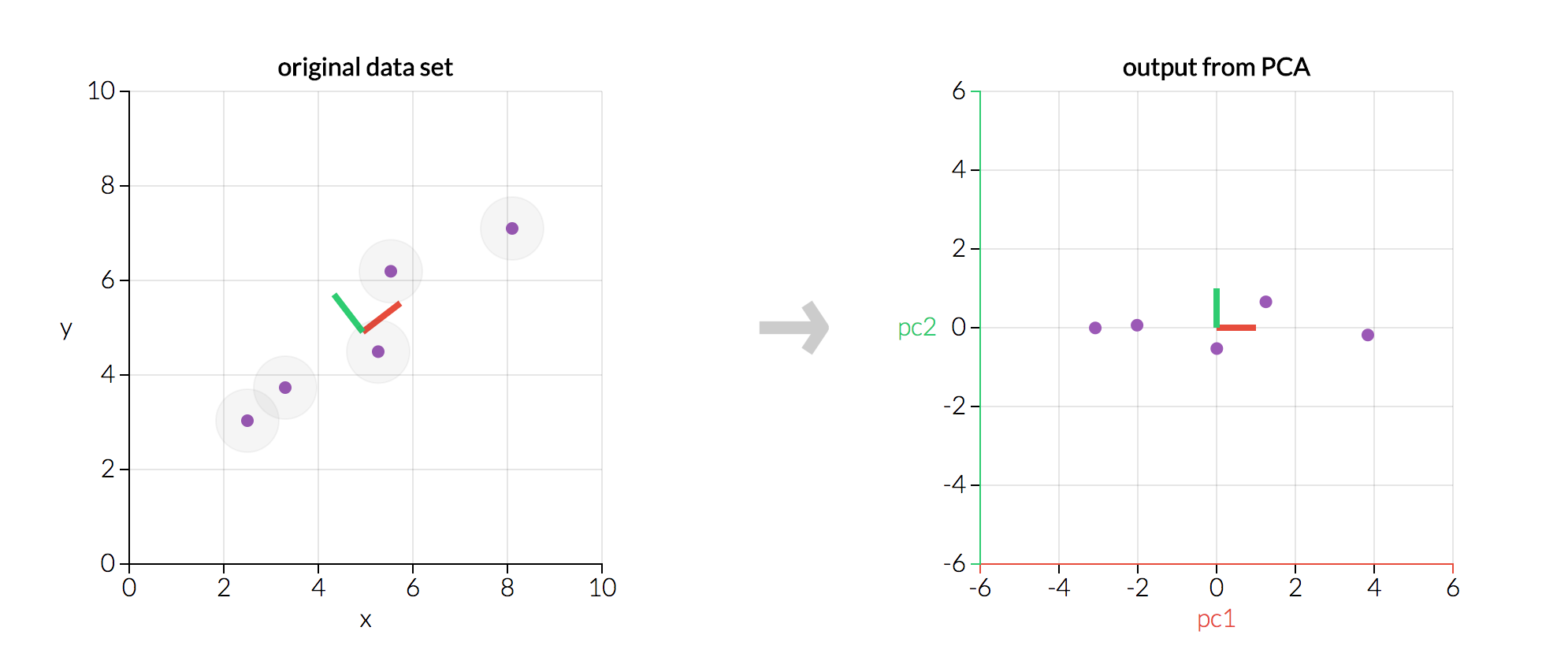/  Top Machine learning interview questions and answers   /  What do you mean by Principal Component Analysis?## What do you mean by Principal Component Analysis?

Principal component analysis is a technique for feature extraction so, it combines our input variables in a specific way, then we can drop the “least important” variables while still retaining the most valuable parts of all of the variables.  PCA does not select a set of features and discard other features, but it infers some new features, which best describe the type of class from the existing features.

PCA works on eigenvectors and eigenvalues of the covariance matrix, which is the equivalent of fitting those straight, principal-component lines to the variance of the data. Because eigenvectors trace the principal lines of force, in other words, PCA determines the lines of variance in the dataset which are called as principal components with the first principal component having the maximum variance, second principal component having second maximum variance and so on.

• Reduces Dimensions
• Searches for a linear combination of variables that best separates 2 classes
• Reduces the degree of overfitting
```# Applying PCA function on training

# and testing set of X component

from sklearn.decomposition import PCA

pca = PCA(n_components = 2)

X_train = pca.fit_transform(X_train)

X_test = pca.transform(X_test)

explained_variance = pca.explained_variance_ratio_```